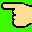\$BBh(B2\$B2s@\$3&%J%&%7%+2q5D(B naucon '97\$BEvF|\$N%9%1%8%e!<%k\$K\$D\$\$\$F(B
Last update :

\$B!{(B8/2(\$BEZ(B) 1st Day

??:??0 meeting time
/* \$B\$\$\$^\$N\$H\$3\$m:E\$7\$b\$N\$N4k2h\$O\$"\$j\$^\$;\$s(B */
12:001st meeting time
JR\$B?7=I1X?7Fn8}2~;%2#(B \$B\$S\$e\$&%W%i%6A0(B
\$B?7=I9bEg20!V!X\$b\$N\$N\$1I1!Y\$N@\$3&E8!W4U>^(B
13:002nd meeting time
JR\$B?7=I1X?7Fn8}2~;%2#(B \$B\$S\$e\$&%W%i%6A0(B
 Lunch time at \$B%(%i%o%s(B? Pre session \$B!V\$b\$N\$N\$1I1!W(B
15:003rd meeting time
JR\$B?7=I1X@>8}(B(\$B%9%P%k%S%kCO2<(B) \$B?7=I\$NL\(B
17:00\$B=I\$X0\F0(B
18:00\$B1c2q(B
 Opening naucon 97 \$B3+2q(B \$B3+2q\$N<-(B(^^? \$B<+8J>R2p\$J\$I(B Video session \$B!VL4MQ3(\$N6q!W(B(\$BLs(B15\$BJ,(B) \$B!V%H%C%W%i%s%J!
20:00\$B1c2q(B(\$B\$=\$N(B2)
 Main session \$B!V\$b\$N\$N\$1I1!W(B
24:00(to be continued ... \$B\$J\$K\$J\$S\$G\$*\$H\$+(B(^^;)

\$B!{(B8/3(\$BF|(B) 2nd Day

8:00\$BD+?)(B
 Closing naucon 97 \$BJD2q(B \$BK\6?@k8@:NBr(B \$B5-G0;#1F(B
10:00\$B2r;6(B

Optional Tour\$B!V\$H\$J\$j\$N%H%H%m?e:L2hE8(B at \$B>.6b0f!W(Bnaucon '97\$B\$N(Bindex\$B\$KLa\$k(B
\$B\$3\$N%Z!<%8\$K4X\$9\$k\$*Ld\$\$9g\$o\$;\$O(B \$B\$_\$o(B (miwa@mt.cs.keio.ac.jp) \$B\$^\$G(B